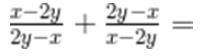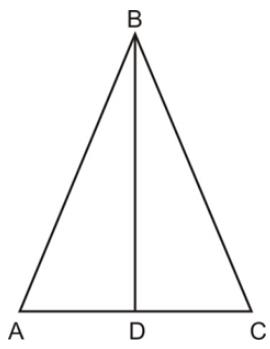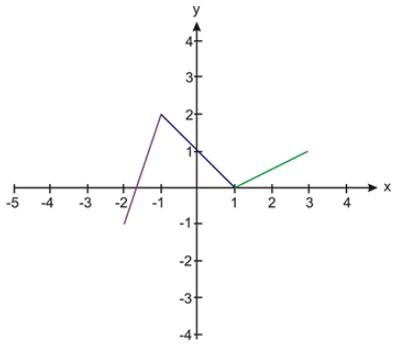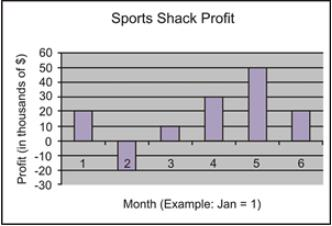Courses

# Test: Problem Solving- 3

## 10 Questions MCQ Test Section-wise Tests for GMAT | Test: Problem Solving- 3

Description
This mock test of Test: Problem Solving- 3 for GMAT helps you for every GMAT entrance exam. This contains 10 Multiple Choice Questions for GMAT Test: Problem Solving- 3 (mcq) to study with solutions a complete question bank. The solved questions answers in this Test: Problem Solving- 3 quiz give you a good mix of easy questions and tough questions. GMAT students definitely take this Test: Problem Solving- 3 exercise for a better result in the exam. You can find other Test: Problem Solving- 3 extra questions, long questions & short questions for GMAT on EduRev as well by searching above.
QUESTION: 1

### A racecar driver has completed 12 1/2 laps of a 50 lap race. What fractional part of the race remains?

Solution:

The driver has 50 - 12 1/2 or 37 1/2 laps to go out of the . Therefore, (37 1/2)/50 is the fractional part of the race remaining. This is equivalent to 3/4, which is answer C.
a. (1/4) This is the fraction of the race that the driver has completed.
b. (1/5) This comes from mistakenly dividing by , the latter number coming from adding both lap amounts. The race is only .
c. Correct
d. (4/5) This comes from taking the fraction in response B and subtracting it from 1.
e. (75/2) This comes from , but that’s how many laps are left - the driver is only in one race, not !

QUESTION: 2

### If M is the set of positive multiples of 2 less than 150 and N is the set of positive multiples of 9 less than 150, how many members are there in M n N?

Solution:

The only elements in both sets are multiples of 2 x 9 or 18. The multiples of 18 less than 150 are 18, 36, 54, 72, 90, 108, 126 and 144. These are the 8 elements that are in the intersection of both sets. Thus, B is the correct response.
a. (0) There are elements that are in both sets.
b. (8) Correct
c. (9) There are 8 elements in both sets, not 9.
d. (18) This is the number that the elements are multiples of.
e. (74) This is the number of multiples of 2 less than 150

QUESTION: 3

### At Bruno’s Video World, the regular price for a DVD is d dollars. How many DVDs can be purchased for x dollars when the DVDs are on sale at 20% off the regular price?

Solution:

Since you have x dollars to spend, you must divide x by the sale price of a CD, which is (100%-20%)d or 80%d . 80%d can be written as a fraction in lowest terms as 4/5d . Therefore, you are dividing x/(4/5d) = 5x/4d. This is answer E.
a. This is the percent of the original price times x.
b. This is the reciprocal of the sale fraction times x.
c. This is the fraction of the original price, which represents the sale price.
d. This comes from incorrectly handling the fraction in the denominator.
e. Correct

QUESTION: 4

If x ≠ 2y, thenSolution:

Both fractions each = -1, so adding -1 twice gives an answer of -2. This is choice E.
a. Both fractions are not equal to (x-2y) , so we do not have like terms.
b. The other parts of the fractions are not eliminated, so this is not possible.
c. Each fraction equals -1, so this result is not possible.
d. Each fraction equals -1, so adding twice gives .
e. Correct

QUESTION: 5

If Dave drove one-third of the distance of his trip on the first day, and 60 miles on the second day, he figured out that he still had 1/2 of the trip to drive. What was the total length, in miles, of his trip?

Solution:

We can model this situation by the equation 1/3x + 60 = 1/2x . Solving for x, we get x=360 , which is answer A.
a. Correct
b. This is the number of miles he drove before he figured out how much of his trip was left.
c. This amount is how much he drove the first day.
d. This is how much he drove the second day.
e. This amount is half of the distance he covered by the end of the second day.

QUESTION: 6

If x2 - y2 = 48, the 2/3(x+y)(x-y) =

Solution:

The difference of two squares (x2-y2) equals 48, and the factors are (x+y)(x-y), so we can just replace both factors in the second expression by 48 and multiply by 2/3 to get 32 . This is choice D.
a. This involves canceling the 3 into the 48 , but forgetting to multiply by 2 .
b. This comes from multiplying 3/2 by 48.
c. This is 48 x 2 , but it was not divided by 3.
d. Correct
e. This is 4/3 times 48.

QUESTION: 7

Eddie is 7 years older than Brian. If Brian is x years old, then how old was Eddie 11 years ago?

Solution:

We can model this by getting Eddie’s age now and then figuring out how old he was 11 years ago. Right now, he is x+7 . Eleven years ago, Eddie was x + 7 -11 = x - 4 years old. This is answer B.
a. The correct calculation should be x+ 7 (to get Eddie’s age now) - 11 , which is x - 4 .
b. Correct
c. This is an incorrect calculation of Eddie’s present age.
d. This response does not relate their ages properly.
e. This also does not express their age relationship properly.

QUESTION: 8

Find the perimeter of lsosceles triangle ABC (below) if mAD = 3 and m < BAC = 55 degrees. Round to the nearest hundredth.Solution:

The first step is to find one of the other sides of the triangle. The easiest is AB. Since we have the side adjacent (next to) the angle we know, we can use cosine. Cosine of an angle = (adjacent)  / (hypotenuse) The setup is cos (55) = 3/(AB). Multiplying AB to the left gives (AB) cos 55 = 3.  Dividing by cos (55) gives  AB = 3/cos(55). Using a calculator gives us  AB = 5.23 Since AB = BC, we know that AB + BC = 10.46 . Also, since AD = DC, we know that AD + DC = 6 Therefore, the sum of all three sides is 16.46.

QUESTION: 9

What is f(2) for the graph of f(x) below?Solution:

Asking for the value of f(2) is just a way of saying “what does y equal when x=2 ?” So you look for when x=2 . On the graph, we have the point (2,1/2) . Note that exact numbers are not provided in the graph, but standard practice indicates that each tick mark is one unit. So we moved 2 tick marks to the right, and then the line crosses through a point on the y axis between 0 and 1. Therefore, f(2)  is equal to 1/2 . Choice B is the only possible choice.

QUESTION: 10

According to the graph below, the greatest change in the profit of the Sports Shack occurred between which two consecutive months?Solution:

A January and February. The profit in January was \$20,000. The profit in February was-\$20,000 . This represents a change in profit of -\$40,000, which is the greatest that the graph shows.

Track your progress, build streaks, highlight & save important lessons and more!

### Similar Content### Related tests